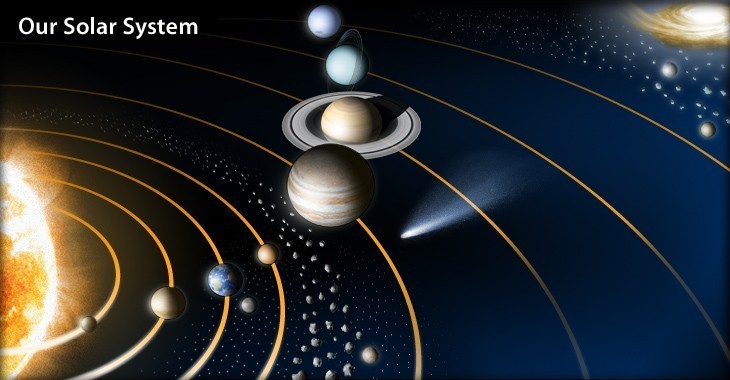# To the Test

In Gravity by Brian Koberlein0 Comments

The popular press has been abuzz about a new proposal to use the solar system to test string theory.  Turns out that is a bit over-hyped.  The actual paper, published in Classical and Quantum Gravity outlines an idea to use the motion of planets and moons to test what is known as the equivalence principle.  The reason string theory gets mentioned is that most string theory models violate the equivalence principle slightly.  Saying this paper proposes a test of string theory is a big stretch.

The basic idea of the equivalence principle was made famous by the (likely mythical) experiment where Galileo dropped things off the leaning tower of Pisa.  The purpose of the experiment was to show that different objects fall at the same rate.  In other words, the rate at which an object falls doesn’t depend upon its mass.

In Newtonian physics, inertial mass is what determines how easy or difficult an object is to move, and gravitational mass determines how strongly a body is attracted to the Earth (or any other gravitational mass).  Since everything near the Earth falls at the same rate, the gravitational mass must be proportional to the inertial mass. That is, the two masses are equivalent, which is the heart of the equivalence principle.

When developing general relativity, Einstein used the equivalence principle as the foundation. His basic argument was that, without some external point of reference, a free-floating observer far from gravitational sources and a free-falling observer in the gravitational field of a massive body each have the same experience. Likewise an observer standing on the surface of a massive body and an observer which uniformly accelerates at a rate equal to the body’s surface gravity have identical experiences. Thus, the free-float and free-fall frames can be considered equivalent. In order to formulate general relativity mathematically, Einstein strengthened this argument to yield what is known as the strong equivalence principle:

The ratio between the inertial mass of a particle and its gravitational mass is a universal constant.

This is the version of the equivalence principle that everyone tries to test, because if it doesn’t hold up, then you’ve shown that general relativity is not the final theory of gravity.  Measuring a violation of the equivalence principle would also open the door to certain quantum gravity models, of which string theory is one approach.

In the paper, the authors start by assuming that the equivalence principle is violated by a small amount, and then calculate how that violation would show up in the motion of planets and moons in the solar system.  Specifically they look for what is known as the Nordtvedt effect, where the relative motion of different masses would shift due to a violation of the equivalence principle.

What they find is that based on current observational data, the inertial and gravitational masses can differ by no more than one part in ten billion.  This is a pretty strong result, but other experiments have validated the equivalence principle to five parts in a hundred trillion, so there is nothing new here.  The paper is interesting because they use planetary motion, but it’s really more of a proof of concept rather than a new scientific result.

Of course none of this really has anything to do with string theory, but it makes for a cool headline.

Paper:  Overduin J, Mitcham J, Warecki Z. Expanded solar-system limits on violations of the equivalence principle. Class. Quantum Grav. 2014;31(1):015001-.